# NI-MCal Calibration Methodology Improves Measurement Accuracy

Publish Date: Oct 08, 2015 | 29 Ratings | 3.83 out of 5 | Print

## Overview

This document details how the National Instruments X Series and M Series data acquisition (DAQ) devices have revolutionized measurement accuracy with NI-MCal. NI-MCal is a software-based calibration algorithm that generates a third-order polynomial to correct for the three sources of voltage measurement error – offset, gain, and nonlinearity. Using software-based measurement corrections, NI-MCal can optimize every selectable range with a unique correction polynomial that hardware-based calibration cannot accommodate.

## Table of Contents

### 1. Defining Accuracy and Error for Calibration

Accuracy
When taking DC voltage measurements, accuracy is the primary concern. Absolute accuracy applies to absolute measurements and describes how consistent a device is compared to an agreed upon standard. Other types of measurements, called relative measurements, can be calculated without any reference to a standard. For example, total harmonic distortion (THD) is a relative measurement. There is no 0% THD standard at the National Institute of Standards and Technology (NIST); rather, THD is a ratio of the power in different frequencies in a signal. Signal-to-noise ratio (SNR) is also a ratio, as are dynamic range, resolution, and number of digits. None of these parameters indicates how accurately a device can measure a 1 V standard.

In addition to being an absolute measurement, accuracy is also a negative term and, as such, has little quantifiable value. To illustrate this concept, consider the physical property of heat. A mechanical engineer will tell you that you cannot add “coldness” to an object. When you make something colder you are really just removing heat (the corresponding positive term to coldness). In the same way, you cannot add accuracy to a measurement, you can only remove error. So to explain how NI-MCal effectively improves accuracy, the remainder of this article focuses on measurement error and ways the technology eliminates or compensates for it.

Error
Voltage measurement error can be broken into three factors: offset, gain and nonlinearity. Figure 1 plots error versus input voltage for a data acquisition device and includes a best-fit line. Offset error is the Y-intercept of this best-fit line. Gain error is the slope of the best-fit line. Nonlinearity error is the worst-case deviation of any point from the best-fit line.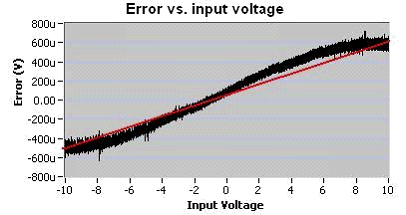Figure 1

### 2. Sources of Measurement Error

All measurement devices roll off an assembly line uncalibrated and full of error. This inaugural error is a sum of the variation of each component used to build the device. Before it is shipped, manufacturers calibrate the device by connecting it to a traceable precision reference and adjusting it until the errors are “calibrated out.” Even this calibration, however, is not permanent, because measurement devices drift according to time and temperature. This phenomenon is why manufacturers specify things such as warm-up time, operating temperature range, and calibration intervals. Operating outside of these limits will cause more drift than what is guaranteed in the device specifications.

### 3. Voltage Reference and Self-Calibration

As soon as a device is calibrated, it starts to drift. In fact, if you went back to a recently calibrated device the next day you might find that it has drifted quite a way from the standard to which it was calibrated. To solve this problem, miniature source standards, called voltage references, are built into measurement devices to add an option for “self-calibration.” Some believe that precision voltage references are used to reduce instrument drift, but this is only partially true. Rather than preventing an instrument from drifting, a voltage reference serves as a portable voltage standard so the instrument can observe and correct for drift by self-calibration. These small references are not as precise as expensive standards used in metrology labs, but are effective and stable enough to keep a device within specifications for a year or two before calibration to an external traceable source is again required.

On most multifunction I/O devices, including legacy NI E Series, self-calibration typically involves making onboard measurements that use an onboard ground source and the onboard voltage reference (often 5 or 7 V). The difference between exactly 0 V and the measured value at ground is used as the offset error. The difference in the slope of the line generated by the two measured points from the expected value is used as the gain error. This rudimentary self-calibration method presents two significant problems: (1) a two-point calibration assumes that the system is linear, and therefore provides no way to account for nonlinearity, and (2) the calibration is performed over a single input range, ignoring the other ranges on the device.

This form of self-calibration is fundamentally flawed because the signal path through the device does not respond linearly; all analog-to-digital converters (ADCs) are, in fact, inherently nonlinear. ADCs are actually specified by their level of nonlinearity. Figure 2 shows an integral nonlinearity (INL) plot, representative of the ones found on nearly every ADC datasheet. The X-axis is the output code of the ADC (18 bit) and the Y-axis is the distance in least significant bits (LSBs) that this code falls from a straight line. As you can see, the INL function for this device is several LSBs in magnitude. This means if you sweep the input voltage from –Full Scale to +Full Scale of the ADC, you observe an error of ±3 LSB from nonlinearity alone. An error of 3 LSB/130000 LSBs gives an error of about 25 ppm of the full scale range, or almost 250 µV for a 10 V input range.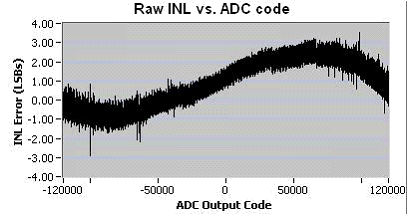Figure 2

During calibration, the nonlinearity of the ADC can make it very difficult to determine what the gain and offset are, as evidenced in Figure 3. The gain error is determined by taking a two-point measurement: one point at ground and one at a positive reference voltage (about 7 V in this case).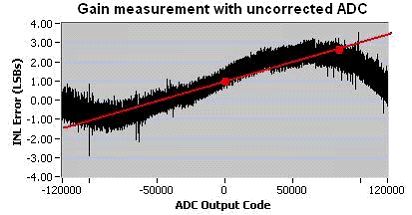Figure 3

Depending on where the two points are taken, you can imagine that quite a bit of variation resulting from gain measurements is made this way. In addition, even small changes in input voltage can cause a lot of variation in measured slope, as can be seen by looking at a zoomed-in portion of the INL plot, as shown in Figure 4. The jagged patterns within the INL function are local nonlinearities resulting from component mismatch within the particular ADC that is being examined and is present in all successive approximation ADCs. Thus, effective calibration must overcome both the large scale S-shaped nonlinearity and the local, jagged nonlinearities.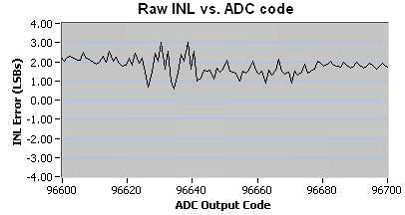Figure 4

### 4. NI-MCal – A New Approach to Calibration

NI-MCal, introduced as a feature on National Instruments M Series devices, takes a unique approach to device self-calibration. In addition to using a new technique in hardware to compensate for measurement error, NI-MCal also uses software to characterize and correct offset, gain, and nonlinearity error. At the heart of the technology is an algorithm that determines a set of third-order polynomial coefficients to accurately translate the digital output of an ADC into voltage data.

### 5. Linearity Correction with NI-MCal

Offset, gain, and nonlinearity error are all referenced to a best-fit line. As a result, gain and offset cannot be accurately characterized until we have determined how the ADC transfer function is shaped around a best-fit line. In simpler terms, this means that to properly characterize total device error, self-calibration must first correct for nonlinearity.

Referencing to Figure 4, it is understood that the INL function exhibits local nonlinearities due to component mismatch within a particular ADC. NI-MCal mitigates error from this local nonlinearity by intentionally introducing noise. When combined with averaging, noise in the signal path smoothes out the INL plot and makes it less jagged. In mathematical terms, the operation is a convolution of the ADCs transfer function with the noise function. NI-MCal takes this idea to the extreme, adding thousands of LSBs of noise or “dither” to the signal before averaging the data to smooth the transfer curve. The result of this smoothing can be seen in Figure 5. All of the INL spikes are gone, leaving only lower-order nonlinearity.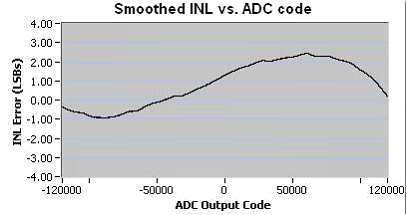Figure 5

To characterize the shape of the curve, NI-MCal uses an onboard, PWM-based digital-to-analog converter (DAC). Through careful layout and design, this DAC is very linear and can be used to characterize the ADC within 2 ppm. NI-MCal does this by sweeping the PWM DAC through the entire ADC input range and then using a 29-point interpolation scheme to “linearize” future readings. The resulting smoothed (from dithering) and linearized (from interpolation) transfer function can be seen in Figure 6.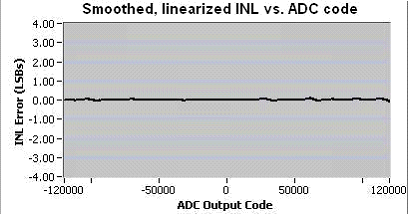Figure 6

The net effect of the linearity correction is that you can make measurements with respect to a best-fit line by dithering, averaging, and applying an interpolation correction scheme. This unique ability of NI-MCal to make measurements with nonlinearity error that is several times better than the raw nonlinearity of the ADC is its greatest strength.

### 6. Offset and Gain Calibration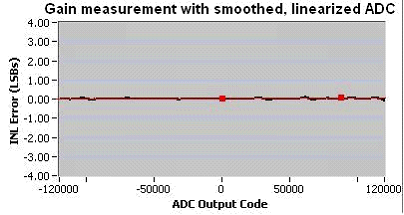Figure 7

NI-MCal uses both the nonlinearity correction and the gain correction to create a third-order polynomial that characterizes all measurement error on the 10 V range.

NI-MCal continues by performing a calibration of each individual input range on the device. Because the voltage reference (typically 5 or 7 V) is outside the measurement range of the smaller (1 V and so on) ranges, the onboard PWM DAC is used in conjunction with the calibrated 10 V range to generate precise voltage references. Thus, for each range, NI-MCal generates a unique, third-order polynomial that converts ADC codes to volts. These scaling coefficients are stored in an onboard memory chip (EEPROM).

### 7. Taking Accurate Measurements with NI-MCal

NI-MCal algorithm executes when a self-calibration function is called from software such as LabVIEW. On a typical GHz PC, NI-MCal takes less than 10 seconds to characterize nonlinearity, gain, and offset, and save the correction polynomials to the onboard EEPROM. Subsequent measurements are scaled automatically by the device driver software before being returned to the user through application software. Unlike other self-calibration schemes, NI-MCal has the unique ability to return calibrated data from every channel in a scan, even if they are at different input ranges. This is because NI-MCal determines, saves, and applies correction polynomials for every input range on the device. Other self-calibration mechanisms use hardware components for data correction, and cannot dynamically load correction functions fast enough to provide accuracy when multiple input ranges are used in a single scan. Instead, NI-MCal uses software for data correction, which can easily load and apply channel-specific correction functions even while scanning at maximum device rates.

NI-MCal performs unlike other self-calibration techniques by correcting for nonlinearity error in addition to applying channel-specific data correction functions for all channels in a scan sequence. By eliminating the limitations of hardware components traditionally used for device error correction and using the power and speed of software and PC processing, NI-MCal raises the bar for measurement accuracy by redefining device self-calibration.

Related Links
Tools to Decrease the Noise Floor
What Is X Series?

### Ratings

Rate this document

Answered Your Question?
Yes No